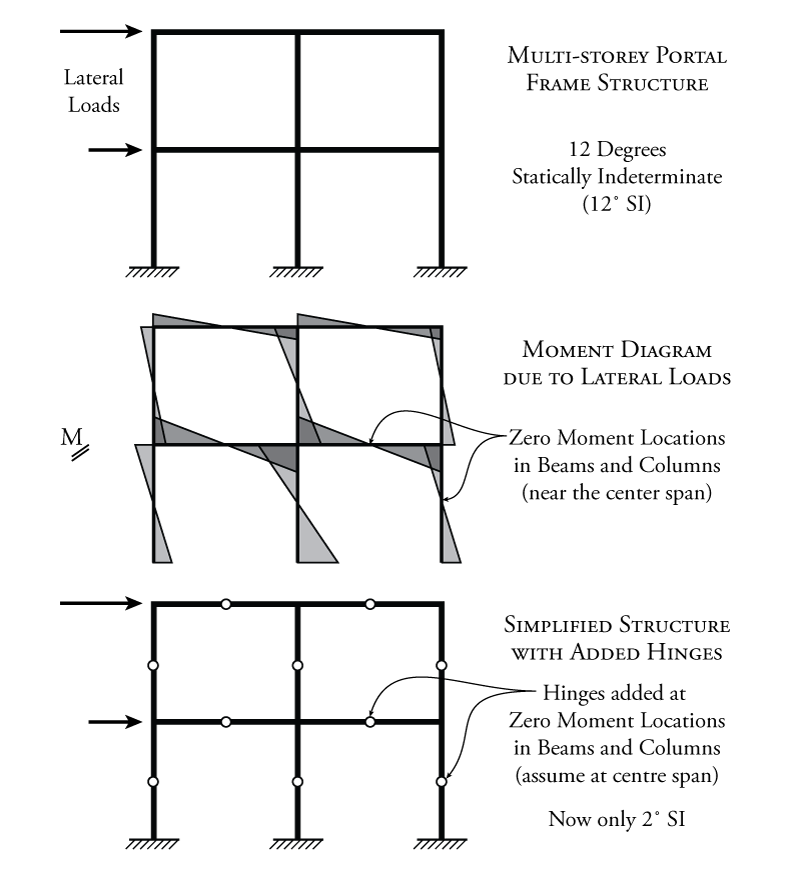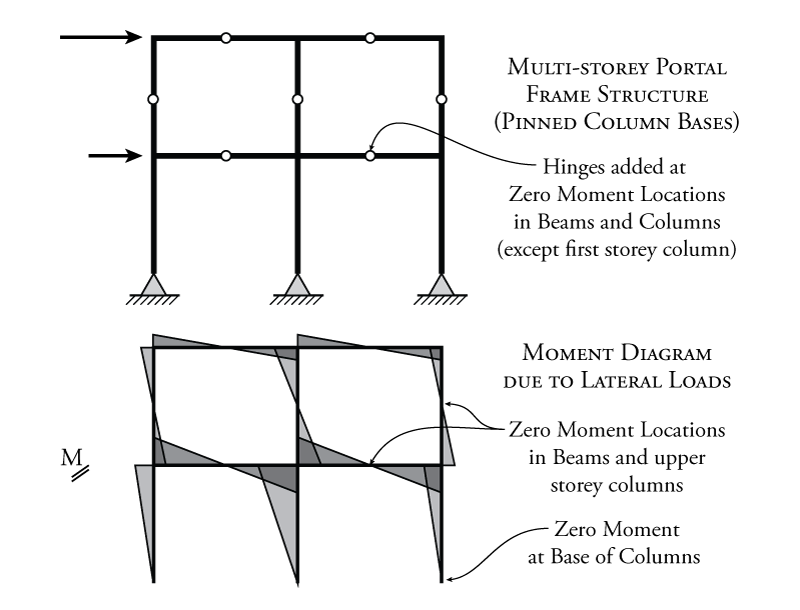# 7.2 Hinge Location Assumption to Reduce the Degree of Indeterminacy

In Figure 7.1, the shape of moment diagram caused by the lateral loads on the building frame is shown in the middle of the figure. Notice that each beam and column are in double-curvature (positive bending at one end, and negative bending at the other). In addition, the distribution of the moments between the two ends of a beam or column is linear from one end to the other. This results in a moment distribution in the beams and columns, which looks like two sets of triangles with a continuous joined hypotenuse. The moments at either end each member are similar, meaning that there is a location of zero moment in the beams and columns somewhere near the centre span.Figure 7.1: Adding Hinges to Approximate the Behaviour of an Indeterminate Portal Frame - Fixed Base

This situation will be more likely if the beams and columns all have similar bending stiffness. This is not necessarily the case in reality, but for most situations where the bending stiffness of the beams and columns are within the same order of magnitude, the zero moment location in the members will be somewhere near the middle of the span or height.

To take advantage of this knowledge, we will assume that the zero moment location is exactly in the middle of the beams and columns. Since we have known zero moment locations in each member, we can add a hinge at those locations as shown in the bottom diagram of Figure 7.1 (since we know that an internal hinge is a location of zero moment in a frame). This gives us one extra equation of condition for each added hinge (see Chapter 2). For the example shown in Figure 7.1, ten hinges are added, one for each member, providing ten equations of condition. This changes our $\ang{12}$ indeterminate structure into a $\ang{2}$ indeterminate structure. This is a vast improvement in our ability to solve the system, but we still cannot solve it using equilibrium because we still have two more unknowns than we have equations.

If the portal frame has pin-ended columns at the base of the structure, as shown in Figure 7.2, then the first storey columns should not have an added hinge because the column will have zero moment at the bottom end, not at the centre of the height. For this configuration, the initial structure (without added hinges), would be $\ang{9}$ indeterminate. With the seven added hinges as shown in Figure 7.2, the structure is left again with just $\ang{2}$ of static indeterminacy.Figure 7.2: Adding Hinges to Approximate the Behaviour of an Indeterminate Portal Frame - Pinned Base

To get rid of the remaining two degrees of static indeterminacy for both the fixed-base and pinned-base frames shown in Figure 7.1 and 7.2, we must make some additional assumptions about the behaviour of the frame. We need a way to figure out some approximate values for some of the remaining unknown forces in the frame. There are two different sets of assumptions that will be discussed here. The first allows us to assume a value for the shear in the columns and is called the Portal Method and the second allows us to assume a value for the axial force in the columns and is called the Cantilever Method.